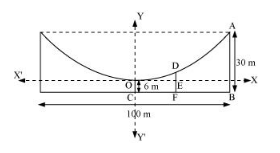# The cable of a uniformly loaded suspension bridge hangs in the form of a parabola.

Question:

The cable of a uniformly loaded suspension bridge hangs in the form of a parabola. The roadway which is horizontal and 100 m long is supported by vertical wires attached to the cable, the longest wire being 30 m and the shortest being 6 m. Find the length of a supporting wire attached to the roadway 18 m from the middle.

Solution:

The vertex is at the lowest point of the cable. The origin of the coordinate plane is taken as the vertex of the parabola, while its vertical axis is taken along the positive y-axis. This can be diagrammatically represented asHere, AB and OC are the longest and the shortest wires, respectively, attached to the cable.

DF is the supporting wire attached to the roadway, 18 m from the middle.

Here, $A B=30 \mathrm{~m}, O C=6 \mathrm{~m}$, and $B C=\frac{100}{2}=50 \mathrm{~m} .$

The equation of the parabola is of the form $x^{2}=4 a y$ (as it is opening upwards).

The coordinates of point A are (50, 30 – 6) = (50, 24).

Since A (50, 24) is a point on the parabola,

$(50)^{2}=4 a(24)$

$\Rightarrow a=\frac{50 \times 50}{4 \times 24}=\frac{625}{24}$

$\therefore$ Equation of the parabola, $x^{2}=4 \times \frac{625}{24} \times y$ or $6 x^{2}=625 y$

The x-coordinate of point D is 18.

Hence, at x = 18,

$6(18)^{2}=625 y$

$\Rightarrow y=\frac{6 \times 18 \times 18}{625}$

$\Rightarrow y=3.11$ (approx)

$\therefore \mathrm{DE}=3.11 \mathrm{~m}$

DF = DE + EF = 3.11 m + 6 m = 9.11 m

Thus, the length of the supporting wire attached to the roadway 18 m from the middle is approximately 9.11 m.